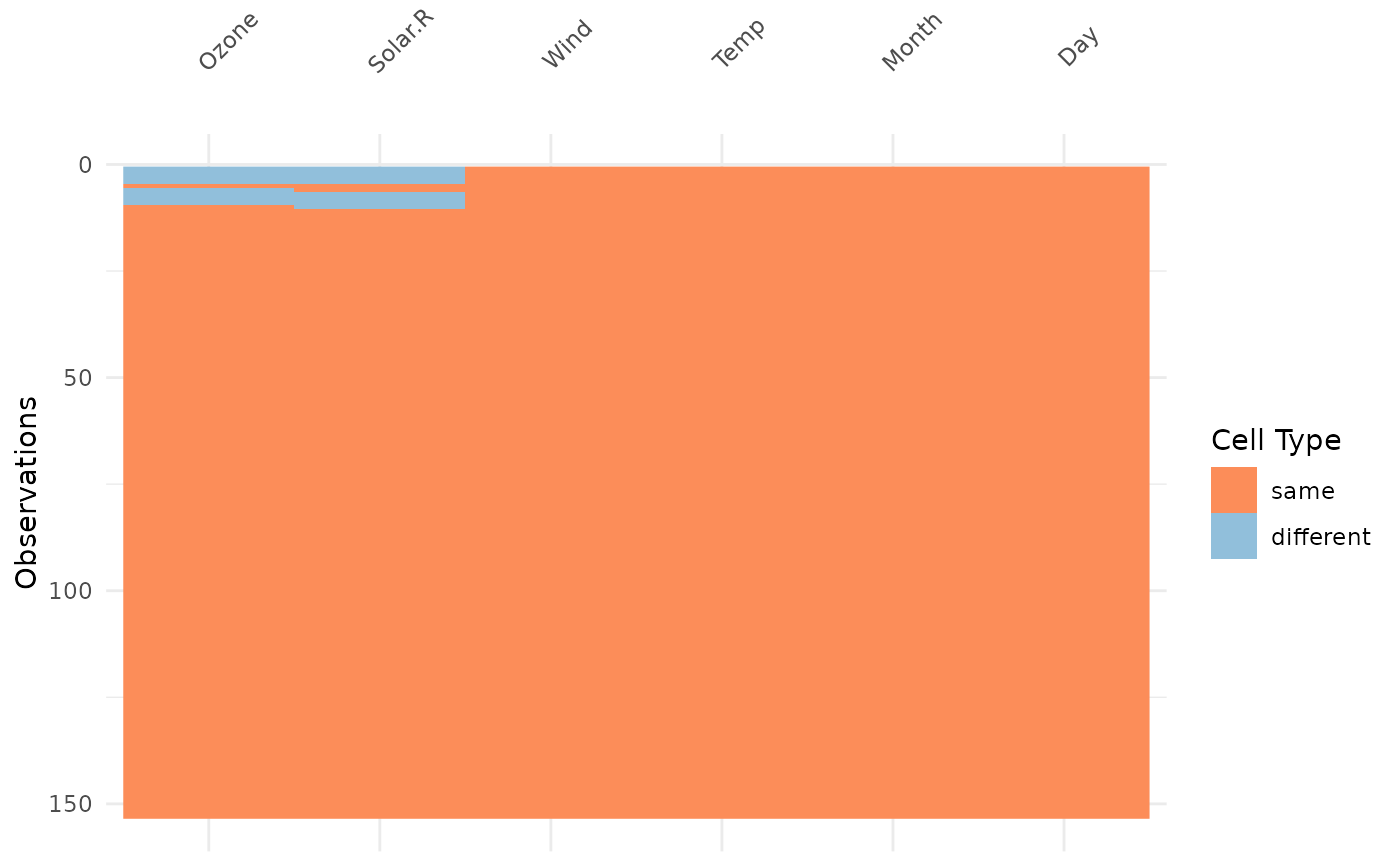`vis_compare`, like the other `vis_*` families, gives an at-a-glance ggplot of a dataset, but in this case, hones in on visualising two different dataframes of the same dimension, so it takes two dataframes as arguments.

## Usage

``vis_compare(df1, df2)``

## Arguments

df1

The first dataframe to compare

df2

The second dataframe to compare to the first.

## Value

`ggplot2` object displaying which values in each data frame are present in each other, and which are not.

`vis_miss()` `vis_dat()` `vis_guess()` `vis_expect()` `vis_cor()`
``````